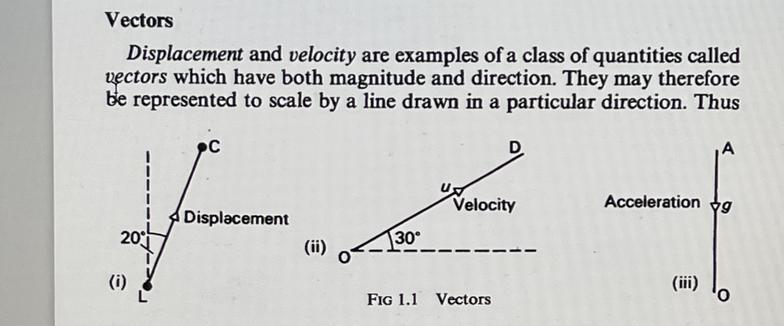MECHANICS,LIGHT,SOUND,HEAT ENERGY

One-dimensional motion

This begins with exploration of ideas of velocity and acceleration.
The average speed of an object is defined as the distance traveled divided by the time elapsed. Velocity is a vector quantity, and average velocity can be defined as the object displaced divided by the time it takes for the displacement.
To find velocity. Divide the total displacement by the total time. In order to find the velocity of the moving object, you will need to divide the change in position by the change in time. Specify the direction moved, and you have the average velocity.
Example 1 The car changed its position by 36 meters over 8 seconds.
Divide 36m by 8s or 36/8 equals 4.5m/sec
​Acceleration, is the rate of change of velocity of an object with respect to time. An object's acceleration is the net result of any and all forces acting on the object, as described by Newton's Second Law. acceleration is metre per second squared (m per s)sq.
A vehicle's capacity to gain speed within a short period of time.
"a Formula One car for example is superior to an Indy car in its acceleration"